The equivalent resistance of the following infinite network of resistances is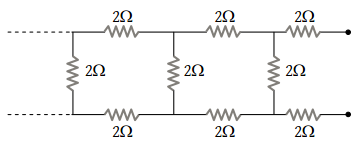(1) Less than 4 Ω

(2) 4 Ω

(3) More than 4 Ω but less than 12 Ω

(4) 12 Ω

Concept Questions :-

Combination of resistors
High Yielding Test Series + Question Bank - NEET 2020

Difficulty Level:

In the figure given below, the current passing through 6 Ω resistor is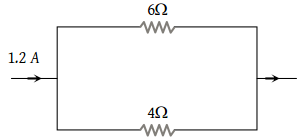(1) 0.40 ampere

(2) 0.48 ampere

(3) 0.72 ampere

(4) 0.80 ampere

Concept Questions :-

Combination of resistors
High Yielding Test Series + Question Bank - NEET 2020

Difficulty Level:

Three equal resistances each of value R are joined as shown in the figure. The equivalent resistance between M and N is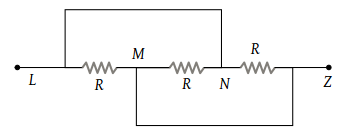(1) R

(2) 2R

(3) $\frac{R}{2}$

(4) $\frac{R}{3}$

Concept Questions :-

Combination of resistors
High Yielding Test Series + Question Bank - NEET 2020

Difficulty Level:

The equivalent resistance between points A and B of an infinite network of resistances each of 1 Ω connected as shown, is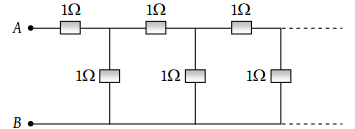(1) Infinite

(2) 2 Ω

(3) $\frac{1+\sqrt{5}}{2}\Omega$

(4) Zero

Concept Questions :-

Combination of resistors
High Yielding Test Series + Question Bank - NEET 2020

Difficulty Level:

In the circuit shown, the point ‘B’ is earthed. The potential at the point ‘A’ is :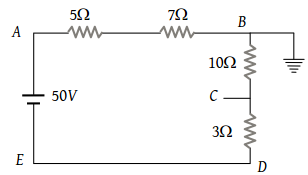(1) 14 V

(2) 24 V

(3) 26 V

(4) 50 V

Concept Questions :-

Combination of resistors
High Yielding Test Series + Question Bank - NEET 2020

Difficulty Level:

In the circuit shown below, the cell has an e.m.f. of 10 V and internal resistance of 1 ohm. The other resistances are shown in the figure. The potential difference ${V}_{A}-{V}_{B}$ is :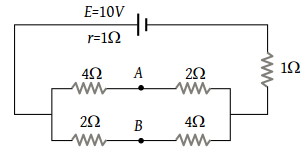(1) 6 V

(2) 4 V

(3) 2 V

(4) –2 V

Concept Questions :-

Combination of resistors
High Yielding Test Series + Question Bank - NEET 2020

Difficulty Level:

A wire of resistance R is cut into ‘n’ equal parts. These parts are then connected in parallel. The equivalent resistance of the combination will be :

(1) nR

(2) $\frac{R}{n}$

(3) $\frac{n}{R}$

(4) $\frac{R}{{n}^{2}}$

Concept Questions :-

Combination of resistors
High Yielding Test Series + Question Bank - NEET 2020

Difficulty Level:

The resistance between the terminal points A and B of the given infinitely long circuit will be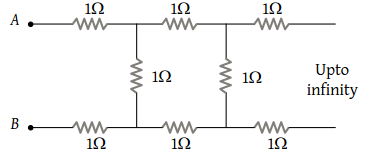(1) $\left(\sqrt{3}-1\right)$

(2) $\left(1-\sqrt{3}\right)$

(3) $\left(1+\sqrt{3}\right)$

(4) $\left(2+\sqrt{3}\right)$

Concept Questions :-

Combination of resistors
High Yielding Test Series + Question Bank - NEET 2020

Difficulty Level:

The current in the given circuit is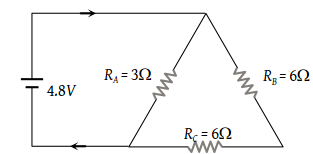(1) 8.31 A

(2) 6.82 A

(3) 4.92 A

(4) 2 A

Concept Questions :-

Combination of resistors
High Yielding Test Series + Question Bank - NEET 2020

Difficulty Level:

What is the current (i) in the circuit as shown in figure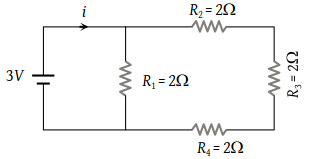(1) 2 A

(2) 1.2 A

(3) 1 A

(4) 0.5 A

Concept Questions :-

Combination of resistors